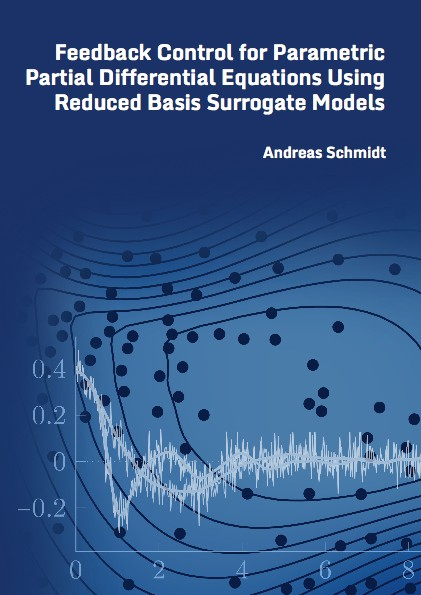Datenbestand vom 25. Mai 2023aktualisiert am 25. Mai 2023

# ISBN 978-3-8439-3805-184,00 € inkl. MwSt, zzgl. Versand

978-3-8439-3805-1, Reihe Mathematik

Andreas Schmidt
Feedback Control for Parametric Partial Differential Equations Using Reduced Basis Surrogate Models

204 Seiten, Dissertation Universität Stuttgart (2018), Softcover, A5

## Zusammenfassung / Abstract

This thesis is about model order reduction methods for parametric and high-dimensional optimal feedback control problems for both linear and nonlinear systems.

In the first part of this work, the reduced basis method for the algebraic Riccati equation is introduced. This method consists of the low-rank factor greedy algorithm for the construction of the basis, a rigorous and highly accurate error estimation theory and a strict offline/online splitting that ensures computational efficiency. The method is then applied to two classical feedback control problems for linear systems: The linear-quadratic regulator and the H2-optimal control problem. Error bounds for the controlled state and cost functional for the linear-quadratic regulator problem guarantee the quality of the approximation in the context of feedback control.

In the second part two techniques for high-dimensional and parametric nonlinear feedback control problems are introduced. The first method combines parametric model order reduction techniques and efficient schemes for solving the parametric Hamilton-Jacobi-Bellman equations that are associated with the optimal control problems. A complete computational workflow is introduced to get an efficient numerical scheme. The second method is a data-driven approach to approximate the value function of the feedback control problems with kernel interpolation techniques.